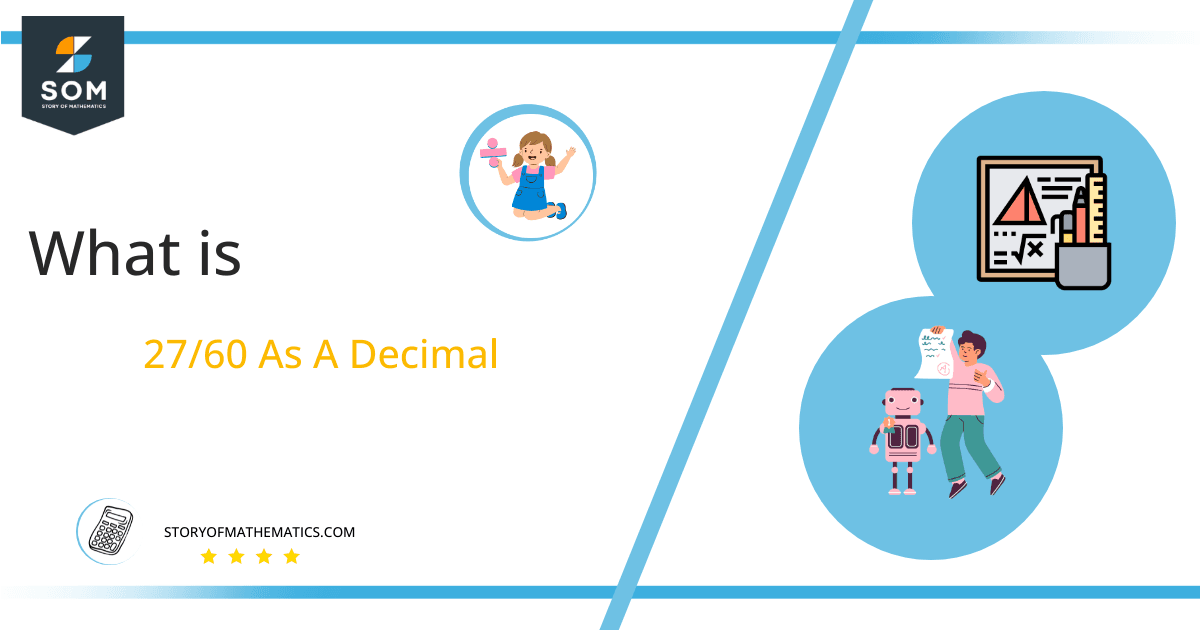# What Is 27/60 as a Decimal + Solution With Free Steps

The fraction 27/60 as a decimal is equal to 0.45.

Fractions are an alternate notation for division. Instead of p $\boldsymbol\div$ q, we write the numeral p/q, where p (the dividend) is called the numerator and q (the divisor) is called the denominator.There are several types of fractions, and 27/60 is a proper fraction (numerator smaller than denominator).Here, we are more interested in the division types that result in a Decimal value, as this can be expressed as a Fraction. We see fractions as a way of showing two numbers having the operation of Division between them that result in a value that lies between two Integers.

Now, we introduce the method used to solve said fraction to decimal conversion, called Long Division, which we will discuss in detail moving forward. So, let’s go through the Solution of fraction 27/60.

## Solution

First, we convert the fraction components, i.e., the numerator and the denominator, and transform them into the division constituents, i.e., the Dividend and the Divisor, respectively.

This can be done as follows:

Dividend = 27

Divisor = 60

Now, we introduce the most important quantity in our division process: the Quotient. The value represents the Solution to our division and can be expressed as having the following relationship with the Division constituents:

Quotient = Dividend $\div$ Divisor = 27 $\div$ 60

This is when we go through the Long Division solution to our problem.Figure 1

## 27/60 Long Division Method

We start solving a problem using the Long Division Method by first taking apart the division’s components and comparing them. As we have 27 and 60, we can see how 27 is Smaller than 60, and to solve this division, we require that 27 be Bigger than 60.

This is done by multiplying the dividend by 10 and checking whether it is bigger than the divisor or not. If so, we calculate the Multiple of the divisor closest to the dividend and subtract it from the Dividend. This produces the Remainder, which we then use as the dividend later.

Now, we begin solving for our dividend 27, which after getting multiplied by 10 becomes 270.

We take this 270 and divide it by 60; this can be done as follows:

270 $\div$ 60 $\approx$ 4

Where:

60 x 4 = 240

This will lead to the generation of a Remainder equal to 270 – 240 = 30. Now this means we have to repeat the process by Converting the 30 into 300 and solving for that:

300 $\div$ 60 $\approx$ 5

Where:

60 x 5 = 300

Finally, we have a Quotient generated after combining the three pieces of it as 0.45, with a Remainder equal to 0.Images/mathematical drawings are created with GeoGebra.